#[資料結構] 使用 C 語言：以連結串列 (Linked List) 為基礎的堆疊 (Stack)

## 堆疊的抽象資料結構``````S is a stack.

sub IsEmpty(S): bool
(Optional) sub IsFull(S): bool
(Optional) sub Size(S): size >= 0
sub Peek(S): data
sub Push(S, data): void
sub Pop(S): data``````

• `IsEmpty(S)`：檢查堆疊是否為空
• `Peek(S)`：檢視堆疊最上方的元素，堆疊大小不變
• `Push(S, data)`：存入新的元素，堆疊大小加 1
• `Pop(S)`：取出堆疊最上方的元素，堆疊大小減少 1

• `IsFull(S)`：檢查堆疊是否已滿
• `Size(S)`：回傳堆疊的大小

## 堆疊型態的公開界面

``````#ifndef STACK_H
#define STACK_H

#ifndef __cplusplus
#include <stdbool.h>
#endif

typedef struct stack_t stack_t;

#ifdef __cplusplus
extern "C" {
#endif

stack_t * stack_new(void);
void stack_delete(void *self);
bool stack_is_empty(const stack_t *self);
int stack_peek(const stack_t *self);
bool stack_push(stack_t *self, const int data);
int stack_pop(stack_t *self);

#ifdef __cplusplus
}
#endif

#endif  /* STACK_H */``````

## 宣告堆疊型態

``````typedef struct node node_t;

struct node {
int data;
node_t *next;
};``````

``````typedef struct stack stack_t;

struct stack {
node_t *top;
};``````

``````typedef struct node node_t;    /*  1 */
typedef node_t * node_p;       /*  2 */

struct node {                  /*  3 */
int data;                  /*  4 */
node_p next;               /*  5 */
};                             /*  6 */

typedef struct stack stack_t;  /*  7 */

struct stack {                 /*  8 */
node_p top;                /*  9 */
};                             /* 10 */``````

## 堆疊的建構函式

``````stack_t * stack_new()
{
stack_t *s = malloc(sizeof(stack_t));
if (!s)
return s;

s->top = NULL;

return s;
}``````

`malloc()` 呼叫實際上是有可能失敗的，故需撰寫錯誤處理相關的程式碼。這裡在 `malloc()` 呼叫失敗時，回傳空的指標。

## 堆疊的解構函式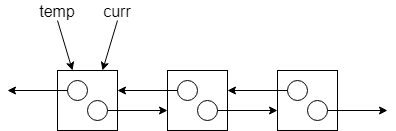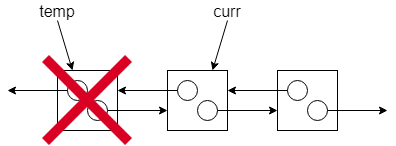``````void stack_delete(void *self)
{
if (!self)
return;

node_t *curr = ((stack_t *) self)->top;
while (curr) {
node_t *temp = curr;
curr = curr->next;
free(temp);
}

free(self);
}``````

## 檢查堆疊是否為空

``````bool stack_is_empty(const stack_t *self)
{
assert(self);

return !(self->top) ? true : false;
}``````

## 檢視堆疊頂端的資料但不取出

``````int stack_peek(const stack_t *self)
{
assert(!stack_is_empty(self));

return self->top->data;
}``````

## 將資料推入堆疊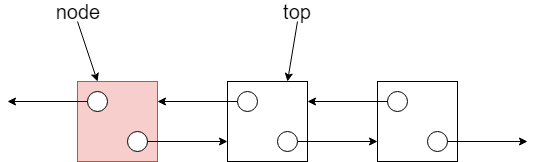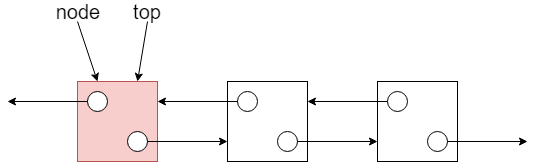``````bool stack_push(stack_t *self, int data)
{
node_t *n = node_new(data);
if (!n) {
return false;
}

n->next = self->top;
self->top = n;

return true;
}``````

``````static node_t * node_new(const int data)
{
node_t *n = malloc(sizeof(node_t));
if (!n) {
return n;
}

n->data = data;
n->next = NULL;

return n;
}``````

## 將資料從堆疊取出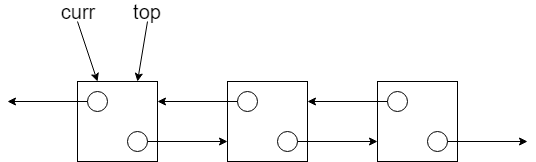`top` 移往下一個節點後，就可以將 `curr` 指向的節點釋放掉：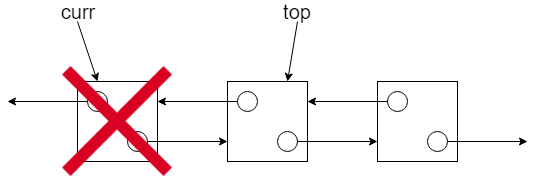``````int stack_pop(stack_t *self)
{
assert(!stack_is_empty(self));

node_t *temp = self->top;
int popped = temp->data;

self->top = temp->next;
free(temp);

return popped;
}``````

## 在演算法上的效率

• `IsEmpty``O(1)`
• `Peek``O(1)`
• `Push``O(1)`
• `Pop``O(1)`